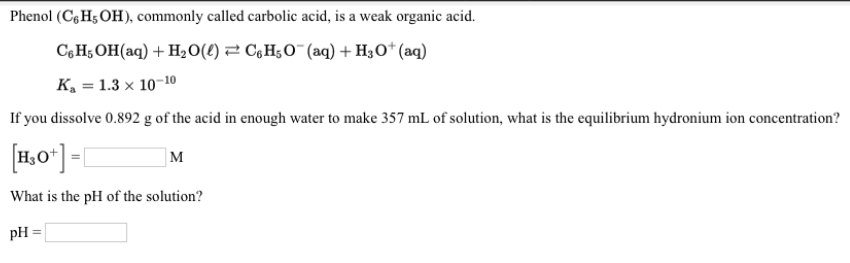# Phenol (C6H5OH), commonly called carbolic acid, is a weak organic acid. C6H5OH(aq) + H2O(ℓ) ⇌ C6H5O^-(aq) + H3O^+(aq) Ka = 1.3 x 10^-10 If you dissolve 0.892 g of the acid in enough water to make 357 mL of solution, what is the equilibrium hydronium ion concentration? What is the pH of the solution?